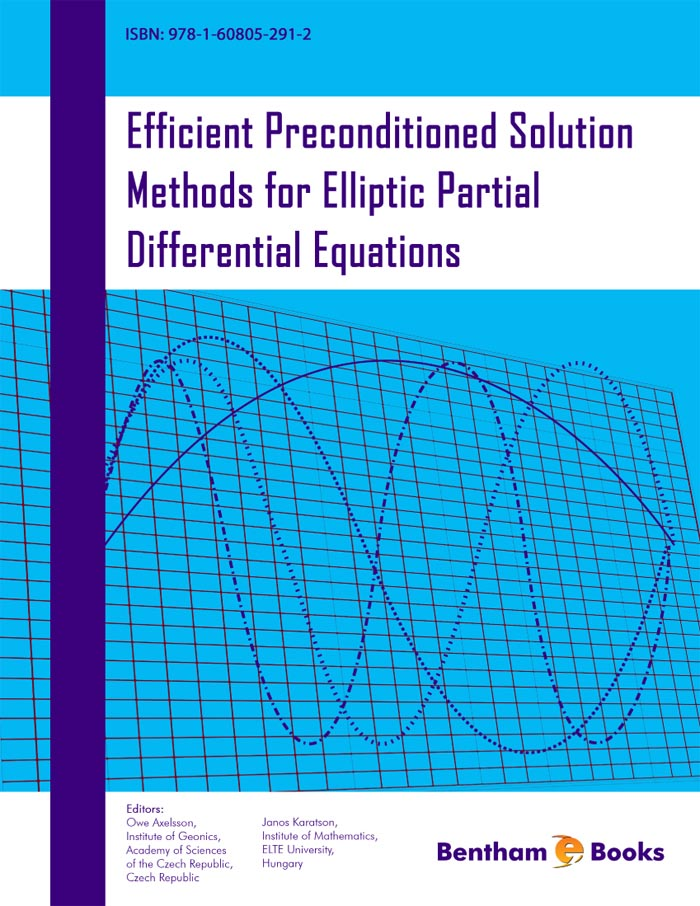### Automatic Construction of Sparse Preconditioners for High-Order Finite Element Methods

#### Abstract

&lt;p&gt;High-order finite elements are necessary for many large scale scientific computing problems because either long time integrations need high accuracy at each time step or modelling certain physics requires the use of high-order finite elements. To reduce the costs of high-order finite elements, robust and efficient linear solvers with minimal memory requirements are crucial. This implies the need for a robust and efficient preconditioner. In the 1980s, Orszag [J. Comp. Phys, 37, 70 (1980)] showed that an inexpensive preconditioner can be defined based on a matrix that is generated from rediscretizing the finite element problem on a finer mesh using low-order finite elements. The matrix was sparse and its inverse proved to be an effective preconditioner.&lt;/p&gt; &lt;p&gt;In this work, we describe a similar sparse preconditioner. However, this preconditioner does not require the user to rediscretize the problem, but only to provide access to the high-order element stiffness matrices. The preconditioner is then an approximate inversion of this sparse matrix with algebraic multigrid, or any other robust method. The sparse matrix is constructed by representing the high-order element stiffness matrices with a sparse approximation generated using a least-squares approach. The least-squares approach aims to accurately represent the low-order modes of the highorder element stiffness matrix. We compare results to Orszag&#039;s approach, where the inverse of the sparse matrix is approximated with a single V-cycle of algebraic multigrid, and to a naive approach that uses a single V-cycle of algebraic multigrid, built from the original high-order system, as the preconditioner.&lt;/p&gt;

Total Pages: 84-102 (19)